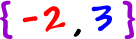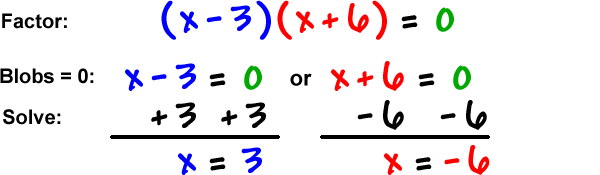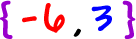Do these answers both work in our original equation? Let's check:They both work, so they are both solutions to the equation. They are really a set of solutions, so we put them together in set brackets:

 The official answer is(No, this is NOT an ( x , y ) point like ( -2, 3 ) .)

Let's do another one:

Solve